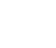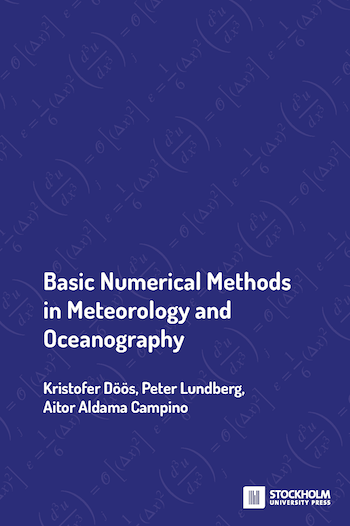Part of# Basic Numerical Methods in Meteorology and Oceanography

### Kristofer Döös, Peter Lundberg, Aitor Aldama Campino

The purpose of this book is to provide an introduction to numerical modelling of the ocean and the atmosphere. It originates from courses given at Stockholm University and is intended to serve as a textbook for students in meteorology and oceanography with a background in mathematics and physics.

Focus is on numerical schemes for the most commonly used equations in oceanography and meteorology as well as on the stability, precision and other properties of these schemes. Simple equations capturing the properties of the primitive equations employed in models of the ocean and atmosphere will be used.

These model equations are solved numerically on a grid by discretisation, the derivatives of the differential equations being replaced by finite-difference approximations. The focus will be on the basic numerical methods used for oceanographic and atmospheric modelling. These models are based on the Navier-Stokes equations (including the Coriolis effect) and a tracer equation for heat in both the atmosphere and ocean and tracer equations for humidity and salt in the atmosphere and ocean, respectively. A coupled atmospheric and oceanic general circulation model represents the core part of an Earth System climate model.

The book starts by presenting the most common types of partial differential equations and finite difference schemes used in meteorology and oceanography. Subsequently the limitations of these numerical schemes as regards stability, accuracy, presence of computational modes and accuracy the computationally determined phase speed are discussed.

The shallow-water equations are discretised for different spatial grids and friction and diffusion terms are introduced. Hereafter implicit and semi-implicit schemes are discussed as well as the semi-Lagrangian technique. Coordinates for atmospheric as well as oceanic models are presented as well as a highly simplified 3D model. A brief description is given of how some atmospheric general circulation models use spectral methods as "horizontal coordinates". Finally, some "pen-and-paper" theoretical exercises and a number of GFD computer exercises are given.

#### View Wikipedia Concepts

These are words or phrases in the text that have been automatically identified by the Named Entity Recognition and Disambiguation service, which provides Wikipedia () and Wikidata () links for these entities.

## Recent Activity

##### How to cite this book
Döös, K et al. 2022. Basic Numerical Methods in Meteorology and Oceanography. Stockholm: Stockholm University Press. DOI: https://doi.org/10.16993/bbs
Döös, K., Lundberg, P. and Aldama Campino, A., 2022. Basic Numerical Methods in Meteorology and Oceanography. Stockholm: Stockholm University Press. DOI: https://doi.org/10.16993/bbs
Döös, K, et al.. Basic Numerical Methods in Meteorology and Oceanography. Stockholm University Press, 2022. DOI: https://doi.org/10.16993/bbs
Döös, K., Lundberg, P., & Aldama Campino, A. (2022). Basic Numerical Methods in Meteorology and Oceanography. Stockholm: Stockholm University Press. DOI: https://doi.org/10.16993/bbs
Döös, Kristofer, Peter Lundberg, and Aitor Aldama Campino. 2022. Basic Numerical Methods in Meteorology and Oceanography. Stockholm: Stockholm University Press. DOI: https://doi.org/10.16993/bbs

##### Export to:This is an Open Access book distributed under the terms of the Creative Commons Attribution 4.0 license (unless stated otherwise), which permits unrestricted use, distribution and reproduction in any medium, provided the original work is properly cited. Copyright is retained by the author(s).